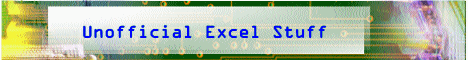Book

Suggestions

Delivered by FeedBurner

Bloglines

1906
2006

OfficeZealot

Scobleizer

TechRepublic

SpyJournalWeb http://www.klippert.comWednesday, March 04, 2009 – Permalink –

Animate Window Size

### So cool!

The following macro has little or no practical computing value, but it can add a "way cool" element when a worksheet is unhidden.
There are three states that a worksheet can be in; Minimized, Maximized, and Normal.

This macro will gradually resize a worksheet from small to Maximized. The worksheet appears to be growing:

```Sub SheetGrow() Dim x As Integer With ActiveWindow .WindowState = xlNormal .Top = 1 .Left = 1 .Height = 50 .Width = 50 For x = 50 To Application.UsableHeight .Height = x Next x For x = 50 To Application.UsableWidth .Width = x Next x .WindowState = xlMaximized End With End Sub```

From AutomateExcel.com:
ActiveWindow.WindowState
(By Mark William Wielgus)

Also fun:

Sub SheetGrow()

Dim x As Integer, xmax As Integer

With ActiveWindow

.WindowState = xlNormal

.Top = 1

.Left = 1

.Height = 50

.Width = 50

If Application.UsableHeight > Application.UsableWidth Then

xmax = Application.UsableHeight

Else

xmax = Application.UsableWidth

End If

For x = 50 To xmax

If x <= Application.UsableHeight Then .Height = x

If x <= Application.UsableWidth Then .Width = x

Next x

.WindowState = xlMaximized

End With

End Sub

# posted by Joerd : 12/30/2005

See all Topics

Labels: , ,

<Doug Klippert@ 3:27 AM